Download PDF文章快速检索 高级检索

1. 辽宁师范大学 计算机与信息技术学院, 辽宁 大连 116081;
2. 南京大学 计算机软件新技术国家重点实验室, 江苏 南京 210093;
3. 辽宁师范大学 数学学院, 辽宁 大连 116081

Linguistic truth-valued intuitionistic fuzzy reasoning with truth-valued qualifications
ZOU Li1,2, TAN Xuewei1, WEN Xin1, LIU Xin3
1. School of Computer and Information Technology, Liaoning Normal University, Dalian 116081, China;
2. State Key Laboratory for Novel Software Technology, Nanjing University, Nanjing 210093, China;
3. School of Mathematics, Liaoning Normal University, Dalian 116081, China
Abstract: In order to help linguistic information be more natural, as well as reduce loss of information while reason-ing, this paper proposes a method for linguistic truth-valued intuitionistic fuzzy reasoning with truth-valued qualifications, by combining with the linguistic truth-valued lattice implication algebra. This paper studies the concepts of linguistic truth-valued intuitionistic fuzzy hesitancy degree, consistency degree, incompatibility degree, and related properties. By calculation of linguistic truth-valued intuitionistic fuzzy consistency degree, truth-value reasoning is reduced. The method of truth-valued qualifications reasoning is given and further detailed steps for reasoning com-putation are demonstrated. An example is given to illustrate that the method is more effective in dealing with linguis-tic truth-valued intuitionistic fuzzy reasoning with both comparability and incomparability.
Key words: intuitionistic fuzzy sets     truth-valued qualifications     hesitancy degree     consistency degree     reasoning

1 预备知识 1.1 直觉模糊集

1.2 六元语言真值直觉模糊代数

1)((hi,t),(hj,f))→((hk,t),(hl,f))=((hmin(3,3-i+k,3-j+l),t),(hmin(3,3-i+l),f));

(2)((hi,t),(hj,f))∨((hk,t),(hl,f))=((hmax( i,k),t),(hmax(j,l),f));

3)((hi,t),(hj,f))∧((hk,t),(hl,f))=((hmin(i,k),t),(hmin(j,l),f));

4)((hi,t),(hj,f))′=((h3-j+1,t),(h3-i+1,f))。

 元 (A,J) (B,K) (C,S) (B,J) (C,K) (C,J) (A,J) (A,J) (B,K) (C,S) (B,J) (C,K) (C,J) (B,K) (A,J) (A,J) (B,K) (A,J) (B,J) (B,J) (C,S) (A,J) (A,J) (A,J) (A,J) (A,J) (A,J) (B,J) (A,J) (B,J) (C,K) (A,J) (B,J) (B,J) (C,K) (A,J) (A,J) (B,J) (A,J) (A,J) (A,J) (C,J) (A,J) (B,J) (C,J) (A,J) (B,J) (A,J)

 元 (A,J) (B,K) (C,S) (B,J) (C,K) (C,J) (A,J) (A,J) (A,J) (A,J) (A,J) (A,J) (A,J) (B,K) (A,J) (B,K) (B,K) (B,J) (B,K) (B,J) (C,S) (A,J) (B,K) (C,S) (B,J) (C,K) (C,J) (B,J) (A,J) (B,J) (B,J) (B,J) (B,J) (B,J) (C,K) (A,J) (B,K) (C,K) (B,J) (C,K) (C,J) (C,J) (A,J) (B,J) (C,J) (B,J) (C,J) (C,J)

 元 (A,J) (B,K) (C,S) (B,J) (C,K) (C,J) (A,J) (A,J) (B,K) (C,S) (B,J) (C,K) (C,J) (B,K) (B,K) (B,K) (C,S) (B,K) (C,K) (C,K) (C,S) (C,S) (C,S) (C,S) (C,S) (C,S) (C,S) (B,J) (B,J) (B,K) (C,S) (B,J) (C,K) (C,J) (C,K) (C,K) (C,K) (C,S) (C,K) (C,K) (C,K) (C,J) (C,J) (C,K) (C,S) (C,J) (C,K) (C,J)
2 语言真值直觉模糊犹豫度

1)没有犹豫的语言真值直觉模糊对有3个，即(A,J),(B,K)和(C,S)；

2)一般犹豫的语言真值直觉模糊对有2个，即(B,J)和(C,K)；

3)非常犹豫的语言真值直觉模糊对有1个，即(C,J)。

1)当m=(A,J)时，i=3且j=3，此时Π(m)=πk=π(3-3)0

m=(B,K)时，i=2且j=2，此时Π(m)=πk=π(2-2)0

m=(C,S)时，i=1且j=1，此时Π(m)=πk=π(1-1)0

2)当m=(B,J)时，i=2且j=3，此时Π(m)=πk=π(3-2)= π1

m=(C,K)时，i=1且j=2，此时Π(m)=πk=π(2-1)1

3)当m=(C,J)时，i=1且j=3，此时Π(m)=πk=π(3-1)2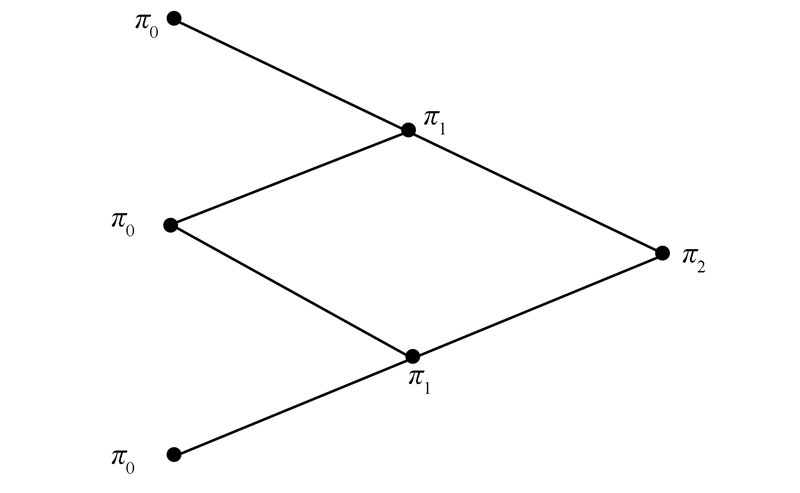图 2 六元语言真值直觉模糊犹豫度结构图Fig. 2 Structure diagram of six-element linguistic truth-valued intuitionistic fuzzy hesitate degree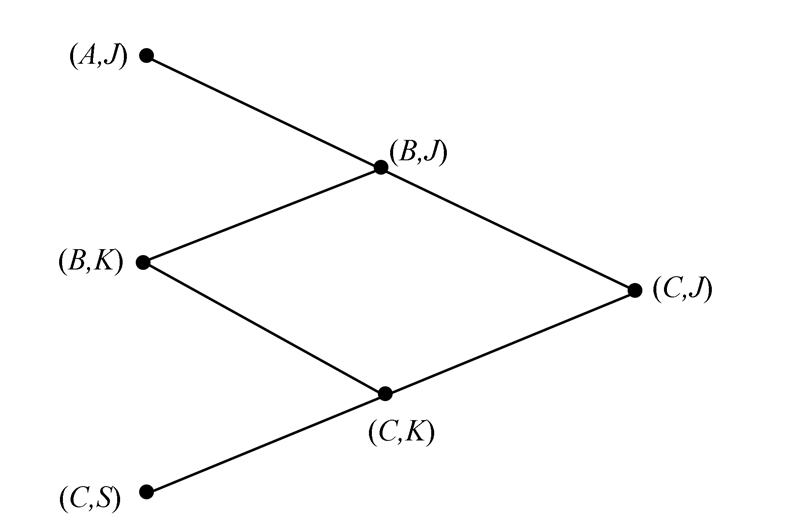图 1 六元语言真值直觉模糊格的结构图Fig. 1 Structure diagram of six-element linguistic truth-valued intuitionistic fuzzy lattice

1)当j不变时，随着i减小，πk增大；

2)当i不变时，随着j增大，πk增大。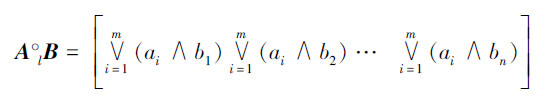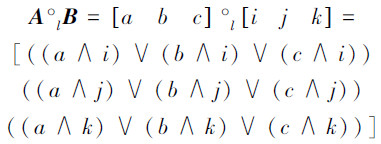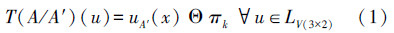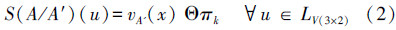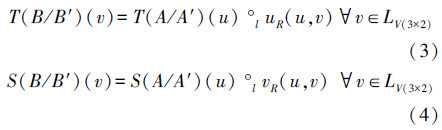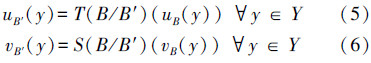3 真值限定的知识表示及推理 3.1 真值限定的语言真值直觉模糊推理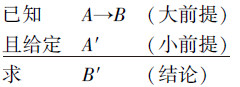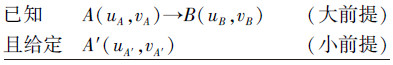3.2 真值限定的语言真值直觉模糊推理算法

1)将命题A(uA,vA),B(uB ,vB),A′(uA′,vA′ )进行简化，而后用向量表示出来；

2)用A(uA,vA)进行限定，分别计算A(uA,vA)与A′(uA,v A′)的语言真值直觉模糊相容度T(A/A′)(u)和语言真值直觉模糊不相容度S(A/A′) (u)；

3)选取六元语言真值直觉模糊蕴涵算子，把大前提A(uA,vA) →B(uB,vB)转化为一个X×Y上的六元语言真值直觉模糊关系R6A(uA,vA) →B(uB,vB)。六元语言真值直觉模糊的蕴涵算子是：((hi,t),(hj,f)) →((hk,t),(hl,f))=((hmin(3,3- i+k,3-j+l),t)，(hmin(3,3-i+l),f)) ；

4)分别将T(A/A′)(u)、S(A/A′)(u)与R6做运算，求出B(uB,vB)与B′(uB′,vB′)的语言真值直觉模糊相容度T(B/B′)(v)和语言真值直觉模糊不相容度S(B/B′)(v)；

5)用B(uB,vB)反限定推导出B′(uB′,vB′)即 uB(y)=T(B/B′)(uB(y))，vB(y)=S(B/B′)(vB(y))。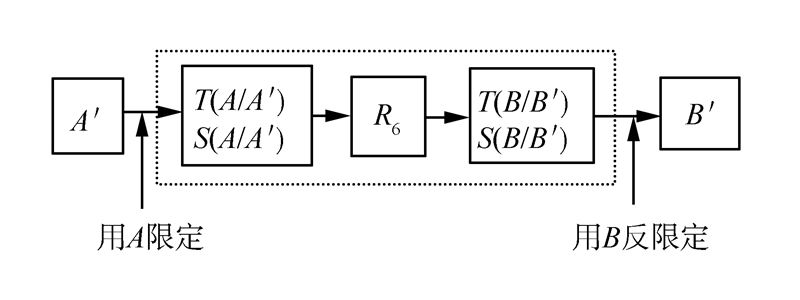图 3 真值限定的语言真值直觉模糊推理过程Fig. 3 True value limit of linguistic truth-valued intuitionistic fuzzy reasoning process

A表示X上的语言真值直觉模糊集“水温低”，则

A=(一般真，非常假)/1+(稍微真，非常假)/2+(稍微真，一般假)/3

B表示Y上的语言真值直觉模糊集“开大热水阀”，则

B=(稍微真，稍微假)/1+(稍微真，非常假)/2+(一般真，非常假)/3

1) 将A,B,A′进行简化，然后用向量表示。

A=(B,J)(C,J)(C,K)

B=(C,S)(C,J)(B,J)

A′=(A,J)(B,J)(C,S)

2)计算相容度T(A/A′ )和不相容度S(A/A′)。

T(A/A′)(B)=uA′(1)Θπk=(h3,t)∨π0=(h3,t)=A

S(A/A′)(J)=vA′(1)Θπk=(h3,f)∨π0=(h3,f)=J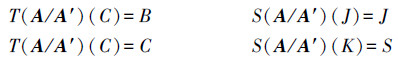S(A/A′)(u)=J/J+J/J+S/K

3) 由六元语言真值直觉模糊关系R6求出 B′B限定下的语言真值。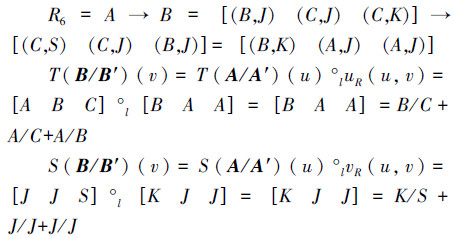4)用B反限定求B′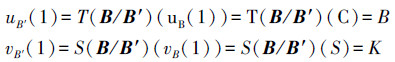B′=(B,K)/1+(A,J)/2+(A,J)/3

B′=(一般真,一般假)/1+(非常真,非常假)/2+(非常真,非常假)/3

4 结束语

  ZADEH L A. Fuzzy sets[J]. Information and Control, 1965, 8(3):338-353.  ATANASSOV K T. Intuitionistic fuzzy sets[J]. Fuzzy Sets and Systems, 1986, 20(1):87-96.  ATANASSOV K T. More on intuitionistic fuzzy sets[J]. Fuzzy Sets and Systems, 1989, 33(1):37-45.  XU Yang, QIN Keyun, LIN Jun, et al. L-valued propositional logic Lvpl[J]. Information Sciences, 1999, 114(1-4):205-235.  徐扬. 格蕴涵代数[J]. 西南交通大学学报, 1993(1):20-27.XU Yang. Lattice implication algebra[J]. Journal of Southwest Jiaotong University, 1993(1):20-27.  夏佩伦. 不确定性推理方法研究[J]. 火力与指挥控制, 2010, 35(11):87-91. XIA Peilun. Comments on techniques for inference with uncertainty[J]. Fire Control & Command Control, 2010, 35(11):87-91.  陈图云, 孟艳平. 模糊集相似度限定推理方法[J]. 工程数学学报, 2005, 22(2):346-348. CHEN Tuyun, MENG Yanping. The reasoning method by fuzzy set similarity degree[J]. Chinese Journal of Engineering Mathematics, 2005, 22(2):346-348.  雷英杰, 王宝树, 路艳丽. 基于直觉模糊逻辑的近似推理方法[J]. 控制与决策, 2006, 21(3):305-310. LEI Yingjie, WANG Baoshu, LU Yanli. Approximate rea-soning method based on intuitionistic fuzzy logic[J]. Control and Decision, 2006, 21(3):305-310.  雷英杰, 汪竞宇, 吉波, 等. 真值限定的直觉模糊推理方法[J]. 系统工程与电子技术, 2006, 28(2):234-236.LEI Yingjie, WANG Jingyu, JI Bo, et al. Technique for in-tuitionistic fuzzy reasoning with truth qualifications[J]. Sys-tems Engineering and Electronics, 2006, 28(2):234-236.  王毅, 雷英杰. 基于直觉模糊逻辑的插值推理方法[J]. 系统工程与电子技术, 2008, 30(10):1944-1948. WANG Yi, LEI Yingjie. Techniques for interpolation rea-soning based on intuitional fuzzy logic[J]. Systems Engineering and Electronics, 2008, 30(10):1944-1948.  赖家骏, 徐扬. 基于语言真值格值一阶逻辑的不确定性推理的语法[J]. 模糊系统与数学, 2011, 25(2):1-60. LAI Jiajun, XU Yang. Syntax of uncertainty reasoning based on linguistic truth-valued lattice value first-order logic[J]. Fuzzy Systems and Mathematics, 2011, 25(2):1-6.  杨丽, 徐扬. 基于概念格的语言真值不确定性推理[J]. 计算机应用研究, 2009, 26(2):553-554, 576. YANG Li, XU Yang. Linguistic truth-valued uncertainty reasoning based on concept lattice[J]. Application Research of Computers, 2009, 26(2):553-554, 576.  邹丽, 谭雪微, 张云霞. 语言真值直觉模糊逻辑的知识推理[J]. 计算机科学, 2014, 41(1):134-137. ZOU Li, TAN Xuewei, ZHANG Yunxia. Knowledge rea-soning based on linguistic truth-valued intuitionstic fuzzy logic[J]. Computer Science, 2014, 41(1):134-137.  郑宏亮, 徐本强, 邹丽. 一种基于十元格蕴涵代数的知识表示方法[J]. 计算机应用与软件, 2013, 30(1):37-40. ZHANG Hongliang, XU Benqiang, ZOU Li. An approach for knowledge representation based on ten-element lattice implication algebra[J]. Computer Application and Sof-tware, 2013, 30(1):37-40.  张云霞, 崔晓松, 邹丽. 一种基于十八元语言值模糊相似矩阵的聚类方法[J]. 山东大学学报, 2013, 43(1):1-7. ZHANG Yunxia, CUI Xiaosong, ZOU Li. A clustering method based on 18-element linguistic-valued fuzzy similar matrix[J]. Journal of Shandong University, 2013, 43(1):1-7.  孙芳, 张凤梅, 邹丽, 等. 基于六元格值命题逻辑的语言真值归结方法[J]. 广西师范大学学报:自然科学版, 2010, 28(3):118-121.SUN Fang, ZHANG Fengmei, ZOU Li, et al. Linguistic truth-valued resolution method based on six-element lattice-valued propositional logic[J]. Journal of Guangxi Normal University:Natural Science Edition, 2010, 28(3):118-121.  邹丽. 基于语言真值格蕴涵代数的格值命题逻辑及其归结自动推理研究[D]. 成都:西南交通大学, 2010:1-160. ZOU Li. Studies on lattice-valued propositional logic and its resolution-based automatic reasoning based on linguistic truth-valued lattice implication algebra[D]. Chengdu, China:Southwest Jiaotong University, 2010:1-160.
DOI: 10.11992/tis.201410006

0

#### 文章信息

ZOU Li, TAN Xuewei, WEN Xin, LIU Xin

Linguistic truth-valued intuitionistic fuzzy reasoning with truth-valued qualifications

CAAI Transactions on Intelligent Systems, 2015, 10(05): 797-802.
DOI: 10.11992/tis.201410006